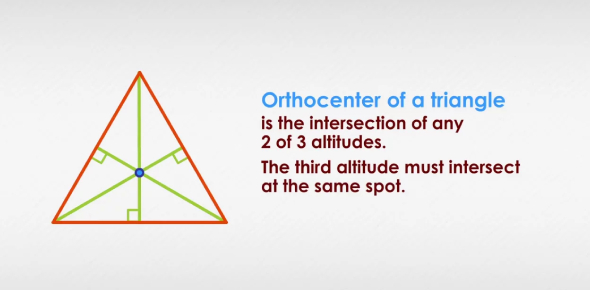# Math Quiz On Triangle Centers! Trivia

17 Questions | Total Attempts: 2380Settings.

• 1.
What is the name of the segment that joins the midpoints of the sides of a triangle?
• A.

Median

• B.

Midsegment

• C.

Vertex

• D.

Centroid

• 2.
Which center is equidistant from the vertices of a triangle?
• A.

Incenter

• B.

Centroid

• C.

Circumcenter

• D.

Orthocenter

• 3.
Which triangle center is the balance point of the triangle?
• A.

Orthocenter

• B.

Incenter

• C.

Centroid

• D.

Circumcenter

• 4.
What is the name of the segment that joins a vertex to the midpoint of the opposite side in a triangle?
• A.

Midsegment

• B.

Median

• C.

Vertex

• D.

Centroid

• 5.
Which center is created by constructing the angle bisectors of a triangle?
• A.

Circumcenter

• B.

Centroid

• C.

Orthocenter

• D.

Incenter

• 6.
What is it called when three or more lines intersect at the same point?
• A.

Concurrent

• B.

Equilateral

• C.

Equiangular

• D.

Conspicuous

• 7.
What is the point of concurrency of the altitudes?
• A.

Incenter

• B.

Centroid

• C.

Circumcenter

• D.

Orthocenter

• 8.
What is the name of the segment drawn from the vertex of a triangle, perpendicular to the opposite side?
• A.

Median

• B.

Midsegment

• C.

Altitude

• D.

Midpoint

• 9.
What is the name of a ray that divides an angle into two equal angles?
• A.

Perpendicular Bisector

• B.

Angle Bisector

• C.

Midpoint

• D.

Median

• 10.
What is the point of concurrency of the medians?
• A.

Incenter

• B.

Orthocenter

• C.

Centroid

• D.

Circumcenter

• 11.
A midsegment's length is _____ that of its parallel side.
• A.

Two-thirds

• B.

Two

• C.

One-half

• D.

One-third

• 12.
What is the point of concurrency of the perpendicular bisectors of a triangle?
• A.

Incenter

• B.

Circumcenter

• C.

Centroid

• D.

Orthocenter

• 13.
What is the point of concurrency of the angle bisectors?
• A.

Incenter

• B.

Centroid

• C.

Circumcenter

• D.

Orthocenter

• 14.
What is the name of a circle that lies outside of the triangle and passes through all vertices of the triangle?
• A.

Inscribed Circle

• B.

Circumscribed Circle

• C.

Orthoscribed Circle

• D.

Round Circle

• 15.
This is which formula?
• A.

Midpoint

• B.

Slope

• C.

Distance

• D.

• 16.
Which formula is this?
• A.

Slope

• B.

Distance

• C.

• D.

Midpoint

• 17.
For what do you use this formula?
• A.

Finding midpoints

• B.

Calculating distance

• C.

Finding slope

• D.

Measuring angle

Related TopicsBack to top